Circle

The Circle command draws a circle from a center location and a radius.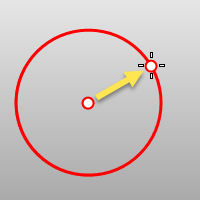Steps

 1 Pick the center location.
 2 Pick a radius location or specify an option.

Command-line options

Deformable

The Deformable option draws a NURBS approximation of the curve will be created with the degree and number of points you specify.Deformable (degree 3) (left); Not deformable (degree 2) (right).

Deformable options

Degree

Specifies the degree of the arc.

When drawing a high-degree arc, the output curve will not be the degree you request unless there is at least one more control point than the degree.

PointCount

Specifies the number of control points.

2Point

The 2Point option draws the circle from the two ends of its diameter.

2Point steps

 1 Pick the start of the diameter.
 2 Pick the end of the diameter.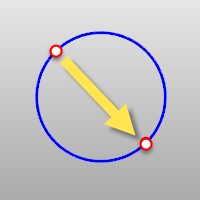3Point

The 3Point option draws a circle through three points on the circumference.

3Point steps

Pick three points that define the circumference.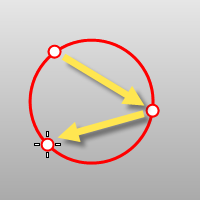3Point option

Specifies the radius and orientation for the circle after two points have been picked.

 1 Pick a location or type a radius value.
 2 Pick a direction to orient the circle.

AroundCurve

The AroundCurve option draws a circle perpendicular to a curve.

AroundCurve steps

Select a curve and pick the center of the circle on the curve and a Radius or Diameter.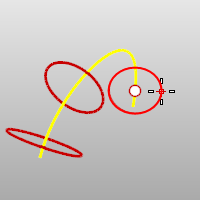FitPoints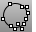The FitPoints option draws a circle by fitting to selected point objects, curve and surface control points, and mesh vertices.

FitPoint steps

Select point objects or control points.

A minimum of three points or control points is required.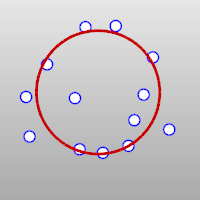Tangent

The Tangent option draws a circle tangent to curves.

Tangent steps

 1 Pick the first tangent location on the first curve.
 2 Pick the second tangent curve, or type a radius.
 3 Pick the third tangent curve, or press Enter to draw the circle from the first and second tangent locations.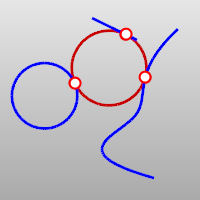Tangent options

FromFirstPoint

Forces the circle or arc to go through the first picked point on the curve instead of allowing the point to slide along the curve.

Point

Allows a point that does not have to be a tangent point on a curve.

The circle is restricted to the specified radius. If a tangent point exists on the second curve that meets the radius requirement, the tangent constraint will appear at that point as you drag the circle.

Vertical

The Vertical option draws a circle perpendicular to the construction plane.

Vertical steps

Pick the center and a radius or diameter.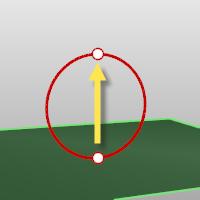Toggles between accepting a radius value and a diameter value.

Pick a location or type a value.

Orientation

Specifies an orientation not on the construction plane

Pick a direction that will be perpendicular to the circle plane.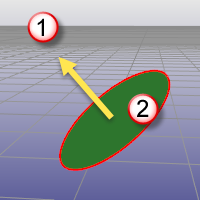Perpendicular direction (1); Circle plane (2).

Circumference

Specifies the circle's circumference.

Type the circumference value at the command line or pick a location to show the circumference length.

Area

Specifies the circle's area.

Type the area value at the command line or pick a location to show the area value